## Sunday, August 30, 2015

### New S3 plot method for rateshift

I just added a new S3 plotting method for objects of class `"rateshift"` created by the phytools function `rateshift`.

This function attempts to fit different Brownian rates of evolutionary change to a user specified number of different 'eras' across the history of the user tree, without specifying when each era should begin & end.

The method is quite simple - and probably interesting to some users - but as I have never published an article describing or using this approach, it probably ranks among the neglected methods of the phytools package.

The new plot method visualizes the different fitted eras on the tree, and includes a color gradient for the fitted rates.

Here's a quick (or, not so quick, if you try to run it) example of what I mean:

``````library(devtools)
install_github("liamrevell/phytools",quiet=TRUE)
library(phytools)
``````
``````## Loading required package: ape
``````

First, let's simulate some data with strong rate shifts over time:

``````set.seed(99)
tree<-pbtree(n=50,scale=100)
x<-sim.rates(tree<-make.era.map(tree,c(0,60,85)),
setNames(c(5,20,1),1:3))
## these are our simulated regimes:
plot(tree,setNames(c("purple","red","blue"),1:3),fsize=0.8,ftype="i",
mar=c(3.1,0.1,0.1,0.1))
axis(1)
``````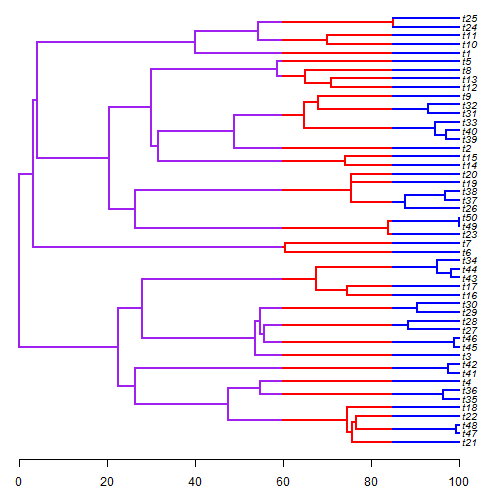Now let's fit our different models:

``````## one rate model (the first two models are easy to fit)
fit1<-rateshift(tree,x,niter=1)
``````
``````## Optimizing. Please wait.
``````
``````fit1
``````
``````## ML 1-rate model:
##  s^2(1)  se(1)   k   logL
## value    7.4779  1.4956  2   -206.1193
##
## This is a one-rate model.
##
## R thinks it has found the ML solution.
``````
``````plot(fit1,fsize=0.7,ftype="i",mar=c(3.1,0.1,0.1,0.1),lwd=3)
axis(1)
``````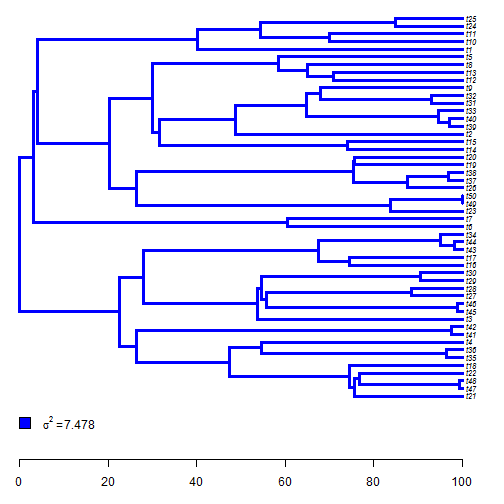``````## two rate model
fit2<-rateshift(tree,x,nrates=2,niter=1)
``````
``````## Optimizing. Please wait.
``````
``````fit2
``````
``````## ML 2-rate model:
##  s^2(1)  se(1)   s^2(2)  se(2)   k   logL
## value    13.8709 3.3576  0.526   0.2121  4   -193.311
##
## Shift point(s) between regimes (height above root):
##  1|2 se(1|2)
## value    88.4271 0.0436
##
## R thinks it has found the ML solution.
``````
``````plot(fit2,fsize=0.7,ftype="i",mar=c(3.1,0.1,0.1,0.1),lwd=3)
axis(1)
``````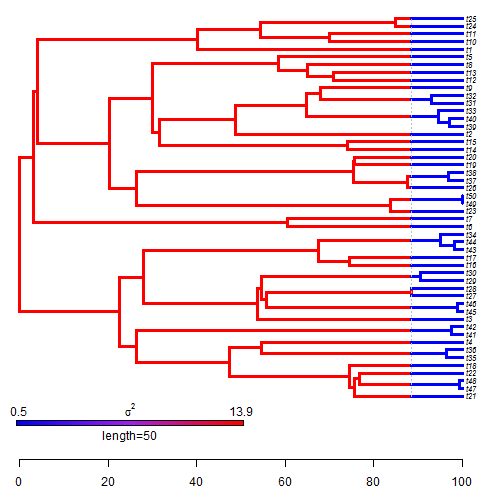``````## three rate model (this was our generating model)
fit3<-rateshift(tree,x,nrates=3,niter=50)
``````
``````## Optimization progress:
## |..................................................|
## Done.
``````
``````fit3
``````
``````## ML 3-rate model:
##  s^2(1)  se(1)   s^2(2)  se(2)   s^2(3)  se(3)   k   logL
## value    3e-04   NaN 17.1992 5.3099  0.5412  0.2269  6   -190.4806
##
## Shift point(s) between regimes (height above root):
##  1|2 se(1|2) 2|3 se(2|3)
## value    48.8427 11.3994 88.4255 0.0819
##
## Optimization may not have converged.
``````
``````plot(fit3,fsize=0.7,ftype="i",mar=c(3.1,0.1,0.1,0.1),lwd=3)
axis(1)
``````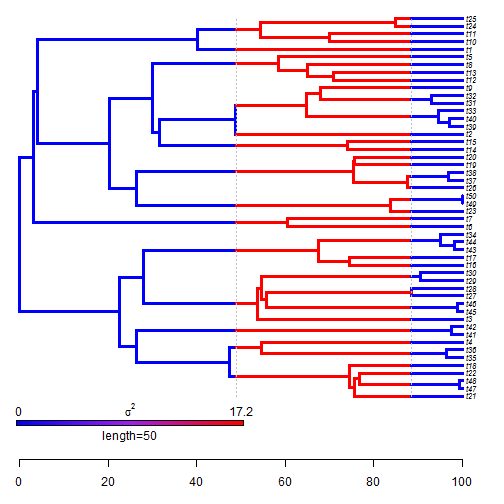``````## four rate model
fit4<-rateshift(tree,x,nrates=4,niter=50)
``````
``````## Optimization progress:
## |..................................................|
## Done.
``````
``````fit4
``````
``````## ML 4-rate model:
##  s^2(1)  se(1)   s^2(2)  se(2)   s^2(3)  se(3)   s^2(4)  se(4)   k   logL
## value    0.0021  NaN 29.1904 12.3032 6.4966  4.7628  0.5255  0.209   8   -189.4281
##
## Shift point(s) between regimes (height above root):
##  1|2 se(1|2) 2|3 se(2|3) 3|4 se(3|4)
## value    53.5568 6.0482  74.1195 0.2341  89.9531 3.9633
##
## Optimization may not have converged.
``````
``````plot(fit4,fsize=0.7,ftype="i",mar=c(3.1,0.1,0.1,0.1),lwd=3)
axis(1)
``````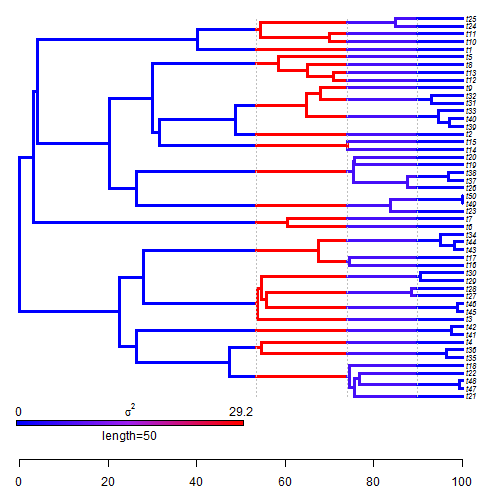The parameter estimates for the three-rate model are quite close - at least in terms of the estimated shift points - to their generating values, which is cool.

We can also compute & compare AIC scores for each model:

``````aics<-setNames(c(AIC(fit1),AIC(fit2),AIC(fit3),AIC(fit4)),
paste(1:4,"-rate",sep=""))
aics
``````
``````##   1-rate   2-rate   3-rate   4-rate
## 416.2387 394.6220 392.9611 394.8562
``````

We see that, in addition to having estimated shift points close to the simulated values, the generating, three-rate model has the best support - although it seems to be quite difficult to achieve convergence for the models with more shift point…. Since the S3 methods are so nice, maybe I should work a little harder at getting the optimization to actually work well!